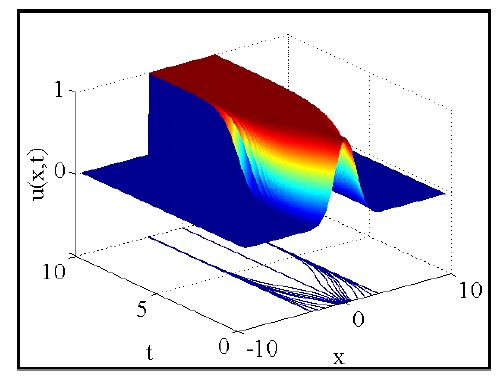### ACM 30220 Partial Differential EquationsI do not currently teach this module. The materials on this page are outdated and are left here for archival purposes only.

Description: In science, partial differential equations arise whenever spatial heterogeneity or evolutionary processes are important. Applications are found in fields as diverse as finance, engineering, physics, and the biosciences. This module is an elementary introduction to the theory of partial differential equations and emphasizes explicit solution techniques for linear and simple nonlinear equations.

Topics and techniques discussed include but are not limited to the following list:

• Fourier series: Finite-dimensional vector spaces, Scalar products, Fourier series, up to and including convergence and term-by term differentiation and integration
• One-dimensional diffusion equation: Physical motivation, solution in terms of sine and cosine series, well-posedness of the solution
• Diffusion with forcing: Diffusion with spatial and temporal forcing, breaking the solution into a homogeneous part and a particular integral
• One-dimensional wave equation: Physical motivation, separation of variables solution, d'Alembert's solution, causality
• Classiffcation of linear first- and second- order PDEs: Classiffcation in terms of the elliptic/parabolic/hyperbolic scheme, classiffcation in terms of the linear/nonlinear dichotomy
• Fourier transforms: Definition and properties, the Dirac delta function, convolution, applications in solving boundary-value problems
• Greens functions: Definition and motivating example for Poisson's equation, general theory for infinite domains in terms of Fourier transforms
• One-dimensional linear advection equation: Physical motivation, solution by the method of characteristics
• One-dimensional Burgers equation with no diffusion: Method of characteristics, breaking waves, Riemann problems
• Burgers equation with diffusion: Proof of regularity, the Cole-Hopf transformation
• Further topics: These may include Greens functions for finite domains, further problems in non-linear first-order PDEs

Learning Outcomes: On completion of this module students should be able to:

1. Compute Fourier series for a given periodic generating function
2. Solve the diffusion equation for a standard set of boundary and initial conditions
3. State and prove the properties of well-posedness for the diffusion problem, and for similar standard linear PDEs
4. Recognize situations where decomposition into homogeneous and particular solutions is necessary, and solve such problems accordingly
5. Classify PDEs as elliptic/parabolic/hyperbolic, and as linear/nonlinear
6. Solve linear inhomogeneous problems using the Green's function technique
7. Solve the one-dimensional wave equation using d'Alembert's formula or separation of variables, depending on the context
8. Understand the notion of causality as applied to the wave equation
9. Solve Burgers equation using the method of characteristics; solve Riemann problems in the same way
10. Obtain a bound on the gradient of the solution in the regularized Burgers problem, apply the Cole-Hopf transformation to the same.

Course documents: I no longer teach this module. However, a complete set of lecture notes relating to an earlier edition of the course is available here: